IF YOU CAN SEE THIS go to /sfa/Nuprl/Shared/Xindentation_hack_doc.html

### Sequents (assertions in Proofs)

There is a form of assertion used in Proofs, designed to facilitate reasoning about Types, Functions, and Propositions. These are called "sequents" (after a similar form introduced by Gentzen). A sequent typically expresses some assertion under hypotheses and under declarations of variables for hypothetical values of specified types. An example is:

1. x :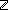2. y :3. x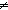y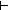x<y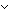y<x

Hypotheses and declarations are normally labelled in the display of a sequent for convenient reference. The place in the sequent filled by "x<yy<x" in this case is called the conclusion of the sequent; sometimes the word "hypothesis" is used broadly to include declarations as well. Some sequent calculuses permit multiple conclusions in a sequent, but Nuprl sequents always have one conclusion. Further, all free variables in the conclusion, or in a hypothesis, or in the type of a declaration, must be declared earlier in the sequent, and no two declarations in a sequent can declare the same variable.

Please, read Functionality Sequents for an introduction to sequents, which is restricted to a special form of sequent, namely, those whose conclusion is of the form "a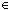A". Here we describe sequents more generally, and with graduated precision.

Version 1.
Roughly, the standard meaning of a Nuprl sequent is that whatever closed expressions one chooses for the declared variables of the sequent, if each resulting (purported) type-expression is actually a type including the value chosen for its declared variable, and each hypothesis is a true proposition, then the conclusion is a true proposition.

Version 2.
Ordinarily one does not need to keep the following complication in mind. It pertains to assumptions as claims about expressions respecting equality, i.e., about Functionality.
The "if" part of the sequent is a little stronger than simply the type expressions being types and having the appropriate chosen values as members, and the hypotheses simply being true. What is further assumed in a sequent is that using any other equal expressions (with respect to the declared types) in place of the chosen expressions results in an equal type for each declaration, and an equal proposition for each hypothesis. Besides the "if" part of the sequent being a little stronger, so is the "then" part: the conclusion must also yield an Equal Proposition (or Equal Type) if different expressions are chosen for the sequent variables.

Version 3.
It is also permitted to use a type expression, say "T", as the conclusion of a sequent. In this case, the sequent is true just when there is some "witness" expression, say "???", that would make the sequent true if the conclusion were replaced by "???T".
This form is especially pertinent to Proof Witness Extraction, and gains further significance when considering Propositions as Types.

For further explanation see Sequents with Type Conclusions.

(March 2001 - sfa )

About: IF YOU CAN SEE THIS go to /sfa/Nuprl/Shared/Xindentation_hack_doc.html